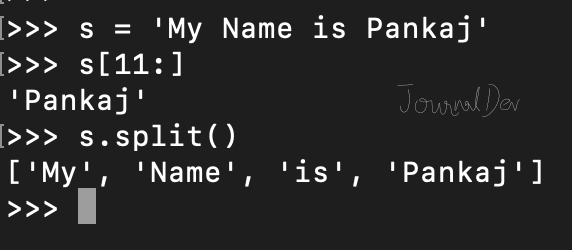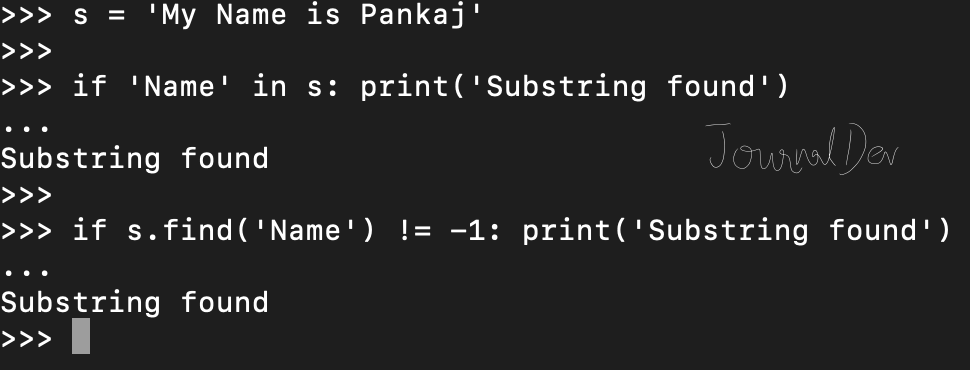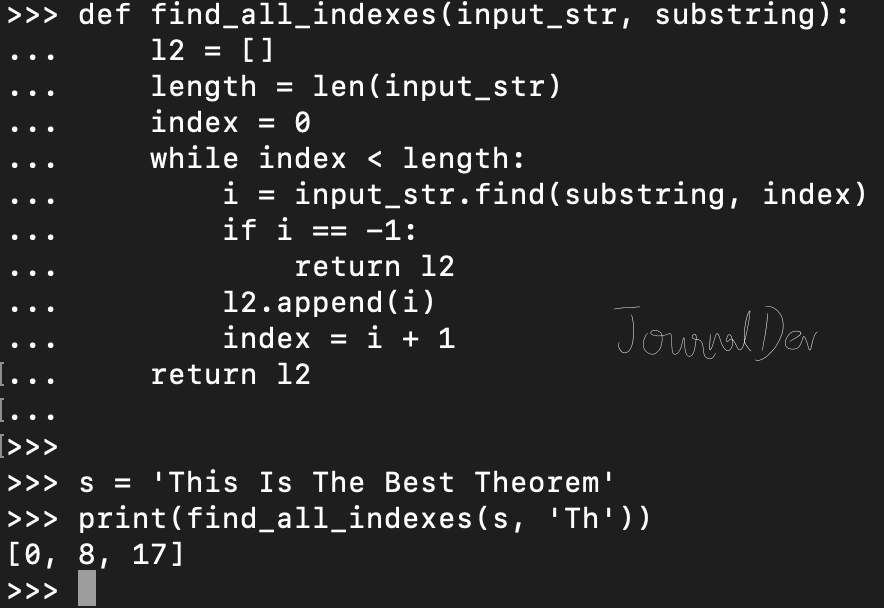# Python String Substring

Published on August 3, 2022By Pankaj
Developer and author at DigitalOcean.While we believe that this content benefits our community, we have not yet thoroughly reviewed it. If you have any suggestions for improvements, please let us know by clicking the “report an issue“ button at the bottom of the tutorial.

A substring is the part of a string. Python string provides various methods to create a substring, check if it contains a substring, index of substring etc. In this tutorial, we will look into various operations related to substrings.

## Python String Substring

Let’s first look at two different ways to create a substring.

### Create a Substring

We can create a substring using string slicing. We can use split() function to create a list of substrings based on specified delimiter.

``````s = 'My Name is Pankaj'

# create substring using slice
name = s[11:]
print(name)

# list of substrings using split
l1 = s.split()
print(l1)
``````

Output:

``````Pankaj
['My', 'Name', 'is', 'Pankaj']
``````### Checking if substring is found

We can use in operator or find() function to check if substring is present in the string or not.

``````s = 'My Name is Pankaj'

if 'Name' in s:
print('Substring found')

if s.find('Name') != -1:
print('Substring found')
``````

Note that find() function returns the index position of the substring if it’s found, otherwise it returns -1.### Count of Substring Occurrence

We can use count() function to find the number of occurrences of a substring in the string.

``````s = 'My Name is Pankaj'

print('Substring count =', s.count('a'))

s = 'This Is The Best Theorem'
print('Substring count =', s.count('Th'))
``````

Output:

``````Substring count = 3
Substring count = 3
``````### Find all indexes of substring

There is no built-in function to get the list of all the indexes for the substring. However, we can easily define one using find() function.

``````def find_all_indexes(input_str, substring):
l2 = []
length = len(input_str)
index = 0
while index < length:
i = input_str.find(substring, index)
if i == -1:
return l2
l2.append(i)
index = i + 1
return l2

s = 'This Is The Best Theorem'
print(find_all_indexes(s, 'Th'))
``````

Output: `[0, 8, 17]`You can checkout complete python script and more Python examples from our GitHub Repository.

### Want to learn more? Join the DigitalOcean Community!

Join our DigitalOcean community of over a million developers for free! Get help and share knowledge in our Questions & Answers section, find tutorials and tools that will help you grow as a developer and scale your project or business, and subscribe to topics of interest.Pankaj

author

Developer and author at DigitalOcean.

#### Still looking for an answer?

program executed,but the list is not printed

- nitin

Thanks for the examples and explanations, are great.

- Jorge